# Pseudo-local tomography

(diff) ← Older revision | Latest revision (diff) | Newer revision → (diff)

Letbe a piecewise smooth, compactly supported function,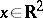. The Radon transform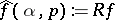is defined by the formula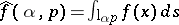, where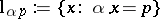is a straight line parametrized by a unit vector,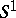is the unit circle inand. By definition,. By local tomographic data one means the values offorandsatisfying the condition, whereis a given point andis a small number. Thus, local tomographic data are the line integrals offor the lines intersecting the "region of interest" , the disc centred atof radius(cf. also Local tomography; Tomography).

It is not possible, in general, to find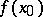from the local tomographic data [a2]. What practically useful information aboutcan one get from these data? Information, very useful practically, is the location of discontinuity curves ofand the sizes of the jumps ofacross these curves.

Pseudo-local tomography solves the problem of finding the above information from the local tomographic data.

This is done by computing the pseudo-local tomography function, introduced in [a2]:(a1)

where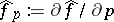. The inversion formula reads: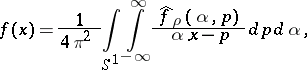(a2)

so that (a1) is based on the following idea: Keep a small neighbourhood of the singular point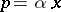in (a1) and neglect the rest of the Cauchy integral in (a1).

By definition, one needs only the local tomographic data to calculate the pseudo-local tomography function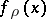.

The basic result is: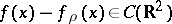is a continuous function [a2], [a1].

Therefore,andhave the same discontinuity curves and the same sizes of the jumps across discontinuities.

It is also proved in [a2] that if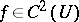, where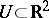is an open set, then the function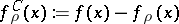has the following properties: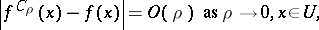(a3)

and the convergence in (a3) is uniform on compact subsets of. If, whereis a smooth discontinuity curve of, then(a4)

Here,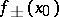are the limiting values ofas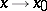from different sides ofalong a path non-tangential to.

If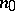is a unit vector normal toat the point, then for an arbitrary,, one has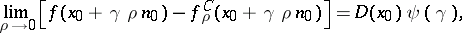where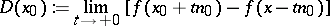andThe function, is monotonically decreasing on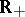,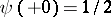,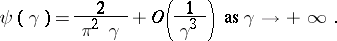If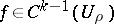, fortheth order derivatives ofexist in, some of them being discontinuous across, andis piecewise-smooth in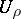, then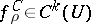.

Other properties ofcan be found in [a2], which also contains a general method for constructing a family of pseudo-local tomography functions, that is, functions which are computable from local tomographic data and having the same discontinuities and the same sizes of the jumps as.

How to Cite This Entry:
Pseudo-local tomography. Encyclopedia of Mathematics. URL: http://encyclopediaofmath.org/index.php?title=Pseudo-local_tomography&oldid=12248
This article was adapted from an original article by A.G. Ramm (originator), which appeared in Encyclopedia of Mathematics - ISBN 1402006098. See original article